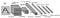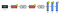# LeNet-5 in Kotlin with TensorFlow

In my previous article, I showed how you can train a linear regression model in Kotlin using Tensorflow API. This time I decided to tackle something a bit more complex, like convolutional networks. In this article I’ll show you how you can train a LeNet model in Kotlin.

## Article Contents:

1. Introduction

# Introduction:

The LeNet-5 architecture was published in 1998, more than 20 years ago, but it remains the cornerstone of all Convolutional Networks. Its building blocks (layers and activation functions) are used in more complex architectures to this day.

“5” — it is very common for the names of neural networks to be derived from the number of convolutional and fully connected layers that they have.

The original paper contains an architecture diagram that is widely known and you probably saw it many times before.LeNet-5 original image

I prefer modern visualization like the one below:Image from the article https://towardsdatascience.com/illustrated-10-cnn-architectures-95d78ace614d

It has 2 convolutional (conv) and 3 fully-connected (dense) layers. It also contains avg-pool blocks that are sub-sampling layers.

This pattern (conv layer + pooling, repeated a few times, plus a few dense layers at the end) became a common pattern in more complex Convolutional Networks and we will see it in the next articles about VGG or AlexNet.

# LeNet-5 layers:

1. Convolution #1. Input = 32x32x1. Output = 28x28x6 `conv2d`

I will use LeNet-5 network to train a model on MNIST dataset that identifies handwritten digits. My architecture will be a little different from the original architecture to reach the local minimum faster.

In Keras, this model looks very simple, but the Keras API is not available yet on JVM, and this model will be presented as a TensorFlow Graph.

Time passed and the original model was eroded by doubts. Recently, classical architecture has undergone cosmetic changes, which I applied in my example on Kotlin. Let me list the main ones:

• ReLU as an activation function instead of Tanh or Sigmoid

# The updated LeNet-4-zaleslaw layers:

1. Convolution #1. Input = 28x28x1. Output = 28x28x32 `conv2d`

First of all, lets define the hyper-parameters and other useful constants, download the MNIST dataset and define placeholders for our data:

`// Hyper-parametersprivate const val LEARNING_RATE = 0.2fprivate const val EPOCHS = 10private const val TRAINING_BATCH_SIZE = 500// Image pre-processing constantsprivate const val NUM_LABELS = 10Lprivate const val NUM_CHANNELS = 1Lprivate const val IMAGE_SIZE = 28Lprivate const val VALIDATION_SIZE = 0private const val SEED = 12Lprivate const val PADDING_TYPE = "SAME"// Tensor namesprivate const val INPUT_NAME = "input"private const val OUTPUT_NAME = "output"private const val TRAINING_LOSS = "training_loss"...val dataset = ...Graph().use { graph ->    val tf = Ops.create(graph)    // Define placeholders    val images = tf.withName(INPUT_NAME).placeholder(        Float::class.javaObjectType,        Placeholder.shape(            Shape.make(                -1,                IMAGE_SIZE,                IMAGE_SIZE,                NUM_CHANNELS            )        )    )    val labels = tf.placeholder(Float::class.javaObjectType)`

What are these numbers in the images tensor shape?

The inputs from MNIST dataset are grayscale images, hence they are in the dimension of [height, width, num_channels] ([28, 28, 1]).

The first dimension is filled with -1 that stands for unknown amount of images for the input of our CNN.

# First Convolutional layer

The typical conv2d layer declaration consist of following steps:

• matrix weights and bias variables declaration;
`val conv1Weights: Variable<Float> =    tf.variable(Shape.make(5L, 5L, NUM_CHANNELS, 32), Float::class.javaObjectType)val conv1Biases: Variable<Float> = tf.variable(Shape.make(32), Float::class.javaObjectType)// Generate random data to fill the weight matrixval truncatedNormal = tf.random.truncatedNormal(    tf.constant(longArrayOf(5, 5, NUM_CHANNELS, 32)),    Float::class.javaObjectType,    TruncatedNormal.seed(SEED))val conv1WeightsInit = tf.assign(       conv1Weights,        tf.math.mul(truncatedNormal,tf.constant(0.1f)))val conv1BiasesInit = tf.assign(    conv1Biases, tf.zeros(        constArray(            tf,            32        ), Float::class.javaObjectType    ))val conv1 = tf.nn.conv2d(    images, conv1Weights, mutableListOf(1L, 1L, 1L, 1L),    PADDING_TYPE)val relu1 = tf.nn.relu(tf.nn.biasAdd(conv1, conv1Biases))`

Ok, but what is conv2d layer doing with input image?

It just transforms the input applying the special convolution function: takes the piece of input pixels and multiples by kernel (special matrix with small size). The weights of this kernel are parameters of the CNN and can be found by the Gradient Descent or other optimizers like Adam or RMSprop.

The correct shape calculation is the hardest thing when you are working with the TensorFlow Graph. I’ll try to give some recommendation and common practices here.

For any 2D convolution layer, assuming it receives input X with dimension of: X — [batch_size, input_height, input_width, input_depth].

Then the weights w of this convolution layer would have a dimension of: w — [filter_height, filter_width, input_depth, output_depth].

This convolution layer outputs a y in dimension of: y — [batch_size, output_height, output_width, output_depth].

The input_depth for this layer is equal to 1 (amount of channels), the output_depth is 32 (amount of wished filters to extract the low-level patterns like lines or pieces of primitive curves).

Need to describe a few numbers in this code snippet:

• the [5;5] is a kernel size (or filter size);

# First pooling layer

Once a feature has been detected, its exact location becomes less important. Only its approximate position relative to other features is relevant. For example, once we know that the input image contains the endpoint of a roughly horizontal segment in the upper left area, a corner in the upper right area, and the endpoint of a roughly ver tical segment in the lower portion of the image, we can tell the input image is a 7. A simple way to reduce the precision with which the position of distinc tive features are encoded in a feature map is to reduce the spatial resolution of the feature map. This can be achieved with a socalled subsampling layers which performs a local averaging and a subsampling, reducing the resolution of the feature map, and reducing the sensitivity of the output to shifts and distortions. .

I prefer MaxPooling to the original AvgPooling.

The declaration is very simple:

`val pool1 = tf.nn.maxPool(    relu1,    tf.constant(intArrayOf(1, 2, 2, 1)),    tf.constant(intArrayOf(1, 2, 2, 1)),    PADDING_TYPE)`

Two intArrays are just packed kernel and stride sizes for each dimension of the input tensor. In reality only middle two (kernel; kernel; strides; strides) play a significant role in TensorFlow CNN training. The values at the edges of the array generally remain filled units.

# Second Convolutional layer and Pooling Layer

This layer is a copy of the previous with a changed amount of input and output filters. The input_depth is 32 due to 32 filters from the first conv2d layer.

The 64 is a new value for filters in the second conv2d layer (could be increased if you wish).

`val truncatedNormal2 = tf.random.truncatedNormal(    tf.constant(longArrayOf(5, 5, 32, 64)),    Float::class.javaObjectType,    TruncatedNormal.seed(SEED))val conv2Weights: Variable<Float> =    tf.variable(Shape.make(5, 5, 32, 64), Float::class.javaObjectType)val conv2WeightsInit = tf.assign(conv2Weights, tf.math.mul(truncatedNormal2, tf.constant(0.1f)))val conv2 = tf.nn.conv2d(    pool1, conv2Weights, mutableListOf(1L, 1L, 1L, 1L),    PADDING_TYPE)val conv2Biases: Variable<Float> = tf.variable(Shape.make(64), Float::class.javaObjectType)val conv2BiasesInit = tf.assign(    conv2Biases, tf.zeros(        constArray(            tf,            64        ), Float::class.javaObjectType    ))val relu2 = tf.nn.relu(tf.nn.biasAdd(conv2, conv2Biases))`

The second MaxPooling layer could be added in the same manner as a previous one.

`val pool2 = tf.nn.maxPool(    relu2,    tf.constant(intArrayOf(1, 2, 2, 1)),    tf.constant(intArrayOf(1, 2, 2, 1)),    PADDING_TYPE)`

# Flatten the 2d input

The next step includes the flattenization of the squared input to the plain vector with the size 3136 (7 * 7 * 64 — shape of the preivous max pooling layer).

The flatten operation is just a reshape along two axis.

`val slice: Slice<Int> = tf.slice(    tf.shape(pool2),    tf.constant(intArrayOf(0)),    tf.constant(intArrayOf(1)))val mutableListOf: MutableList<Operand<Int>> = mutableListOf(slice, tf.constant(intArrayOf(-1)))val flatten = tf.reshape(    pool2,    tf.concat(        mutableListOf,        tf.constant(0)    ))`

The result of applying the flatten operand is the input for the dense layer.

# Dense layers and the output

It’s time for good old fully-connected layers.

In reality, the combination of Flatten operand and first dense layer is not exactly the typical fully-connected layer (in the  it is described as a convolutional layer with kernel 1x1).

Each unit is connected to a 5x5 neighborhood on all 64 features maps (filters).

The dense layer includes:

• weight and bias variable declaration;

Similar to conv2d layer, I agree, but it operates with vectors not matrices and has no special operation to apply to the input like convolution function.

`val truncatedNormal3 = tf.random.truncatedNormal(    tf.constant(longArrayOf(IMAGE_SIZE * IMAGE_SIZE * 4, 512)),    Float::class.javaObjectType,    TruncatedNormal.seed(SEED))val fc1Weights: Variable<Float> =    tf.variable(Shape.make(IMAGE_SIZE * IMAGE_SIZE * 4, 512), Float::class.javaObjectType)val fc1WeightsInit = tf.assign(fc1Weights, tf.math.mul(truncatedNormal3, tf.constant(0.1f)))val fc1Biases: Variable<Float> = tf.variable(Shape.make(512), Float::class.javaObjectType)val fc1BiasesInit = tf.assign(fc1Biases, tf.fill(tf.constant(intArrayOf(512)), tf.constant(0.1f)))val relu3 = tf.nn.relu(tf.math.add(tf.linalg.matMul(flatten, fc1Weights), fc1Biases))`

The second dense layer forms the outputs for 10 classes in multi-classification task:

`val truncatedNormal4 = tf.random.truncatedNormal(    tf.constant(longArrayOf(512, NUM_LABELS)),    Float::class.javaObjectType,    TruncatedNormal.seed(SEED))val fc2Weights: Variable<Float> =    tf.variable(Shape.make(512, NUM_LABELS), Float::class.javaObjectType)val fc2WeightsInit = tf.assign(fc2Weights, tf.math.mul(truncatedNormal4, tf.constant(0.1f)))val fc2Biases: Variable<Float> = tf.variable(Shape.make(NUM_LABELS), Float::class.javaObjectType)val fc2BiasesInit =    tf.assign(fc2Biases, tf.fill(tf.constant(intArrayOf(NUM_LABELS.toInt())), tf.constant(0.1f)))val logits = tf.math.add(tf.linalg.matMul(relu3, fc2Weights), fc2Biases)`

There is no activation function due to special metric that will be used later (it includes the last step with sigmoid activation).

# Training: loss function, gradient descent

To calculate the loss function value I decided to use the special function softmaxCrossEntropyWithLogits on each batch and average it across the whole dataset.

`val batchLoss = tf.nn.softmaxCrossEntropyWithLogits(logits, labels)val loss = tf.withName(TRAINING_LOSS).math.mean(batchLoss.loss(), tf.constant(0))`

After that, we can set up the Gradient Descent manually:

• define learning rate;
`// Define gradientsval learningRate = tf.constant(LEARNING_RATE)val variables = listOf(conv1Weights, conv1Biases, conv2Weights,   conv2Biases, fc1Weights, fc1Biases, fc2Weights, fc2Biases)val gradients = tf.gradients(loss, variables)val variablesGD = variables.mapIndexed { index, variable ->    tf.train.applyGradientDescent(variable, learningRate, gradients.dy(index))}val variablesInit = listOf(conv1WeightsInit, conv1BiasesInit, conv2WeightsInit, conv2BiasesInit, fc1WeightsInit, fc1BiasesInit, fc2WeightsInit, fc2BiasesInit)fun <T, E> T.applyF(f: T.(E) -> T, ls: Iterable<E>) =   ls.fold(this,f)Session(graph).use { session ->    // Initialize graph variables    session.runner()        .applyF(Session.Runner::addTarget, variablesInit)        .run()`

When all variables are ready to be recalculated by Backward Propagation, we run the main training loop for N epochs with yet once more internal cycle by batches during each epoch.

`// Train the graphfor (i in 1..EPOCHS) {    val batchIter: ImageDataset.ImageBatchIterator = dataset.trainingBatchIterator(        TRAINING_BATCH_SIZE    )    while (batchIter.hasNext()) {        val batch: ImageBatch = batchIter.next()        Tensor.create(            longArrayOf(                batch.size().toLong(),                IMAGE_SIZE,                IMAGE_SIZE,                NUM_CHANNELS            ),            batch.images()        ).use { batchImages ->            Tensor.create(longArrayOf(batch.size().toLong(), 10), batch.labels()).use { batchLabels ->                val lossValue = session.runner()                    .applyF(Session.Runner::addTarget, variablesGD)                    .feed(images.asOutput(), batchImages)                    .feed(labels.asOutput(), batchLabels)                    .fetch(TRAINING_LOSS)                    .run().floatValue()                println("epochs: \$i lossValue: \$lossValue")            }        }    }}`

# Evaluation: meet the Accuracy Queen!

Not much of a use to train the model without metric calculation on test dataset.

Let’s form the evaluation TensorFlow graph with Accuracy Metric to evaluate the trained model on the test part of MNIST dataset.

`val prediction = tf.withName(OUTPUT_NAME).nn.softmax(logits)val predicted: Operand<Long> = tf.math.argMax(    prediction, tf.constant(1))val expected: Operand<Long> = tf.math.argMax(labels, tf.constant(1))// Define multi-classification metricval accuracy = tf.math.mean(    tf.dtypes.cast(        tf.math.equal(predicted, expected),        Float::class.javaObjectType    ), constArray(tf, 0))`

Here, we should apply the Softmax activation function to the logits node, because the Softmax could give us the probabilities for image to be image of the given class (for each class).

At the end we run our test data through the model’s tensors without gradient calculations to predict on test data and compare with ground truths.

`val testBatch: ImageBatch = dataset.testBatch()Tensor.create(    longArrayOf(        testBatch.size().toLong(),        IMAGE_SIZE,        IMAGE_SIZE,        NUM_CHANNELS    ),    testBatch.images()).use { testImages ->    Tensor.create(testBatch.shape(10), testBatch.labels()).use { testLabels ->        session.runner()            .fetch(accuracy)            .feed(images.asOutput(), testImages)            .feed(labels.asOutput(), testLabels)            .run().use {                value -> println("Accuracy: " + value.floatValue())              }    }}`

# Conclusion

Happy to see you at the end of this article. The full Kotlin code for this example is available here . The Java version of this code is available here .

Today we reviewed the classic model of handwriting recognition. Of course, to distinguish moving cats from standing 3D dogs, it is not suitable in this form. But the basic concepts and pieces of a programmed computational graph presented in this article may well be reused to write more complex models in Kotlin or Java.

P.S. I’m sure that without a deep understanding of TensorFlow computational graph and manual shape calculation, no matter how good you are with Keras, it would prove challenging to create something really new and ready for production.

Apache Ignite Committer/PMC; Machine Learning Engineer in JetBrains